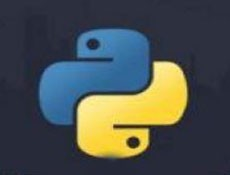# python能判断数字类型吗• silencement   2019-11-11 09:52:28898python中有一个自带的函数isdigit，可以返回某字符串是不是数字。如果是数字，返回True；反之，返回False。

```str1 = '1'
print(str1.isdigit())
str2 = '1.1'
print(str2.isdigit())
str3 = '-1'
print(str3.isdigit())
str4 = 'a'
print(str4.isdigit())```

```True
False
False
False```

python学习网，大量的免费python学习视频，欢迎在线学习！

```def is_number(s):
try:  # 如果能运行float(s)语句，返回True（字符串s是浮点数）
float(s)
return True
except ValueError:  # ValueError为Python的一种标准异常，表示"传入无效的参数"
pass  # 如果引发了ValueError这种异常，不做任何事情（pass：不做任何事情，一般用做占位语句）
try:
import unicodedata  # 处理ASCii码的包
unicodedata.numeric(s)  # 把一个表示数字的字符串转换为浮点数返回的函数
return True
except (TypeError, ValueError):
pass
return False```

```str5 = '八'
print(is_number(str5))```

`True`